# RD Sharma Solutions for Class 10 Maths Chapter 4 Triangles Exercise 4.6

More about the similarity of triangles is discussed in this exercise. Other characteristic properties and areas of two similar triangles are the key concepts covered in the exercise problems. The RD Sharma Solutions Class 10 have all the solutions required for quick reference and preparation for examinations. Further, the students can make use of the RD Sharma Solutions for Class 10 Maths Chapter 4 Triangles Exercise 4.6 PDF provided below.

## RD Sharma Solutions for Class 10 Maths Chapter 4 Triangles Exercise 4.6 Download PDF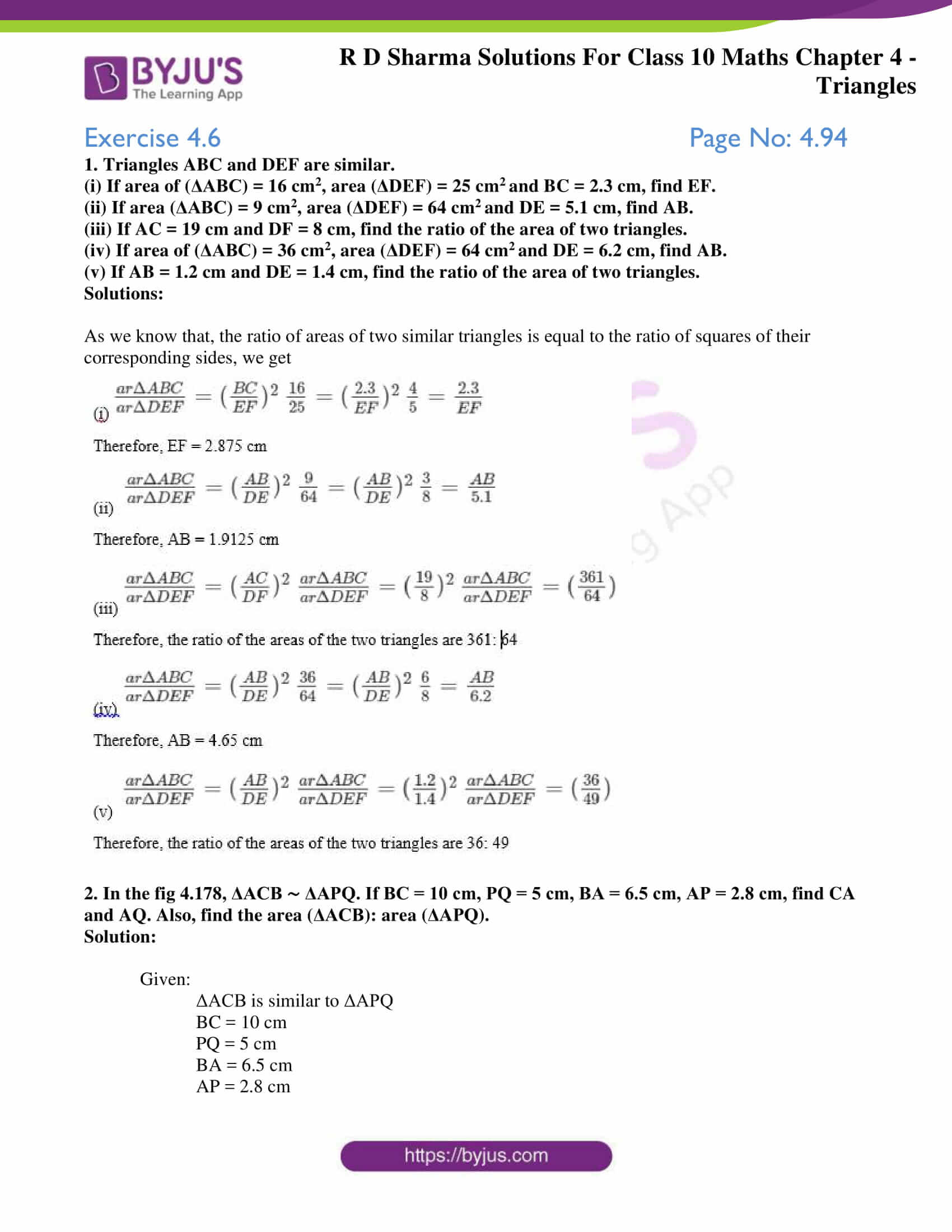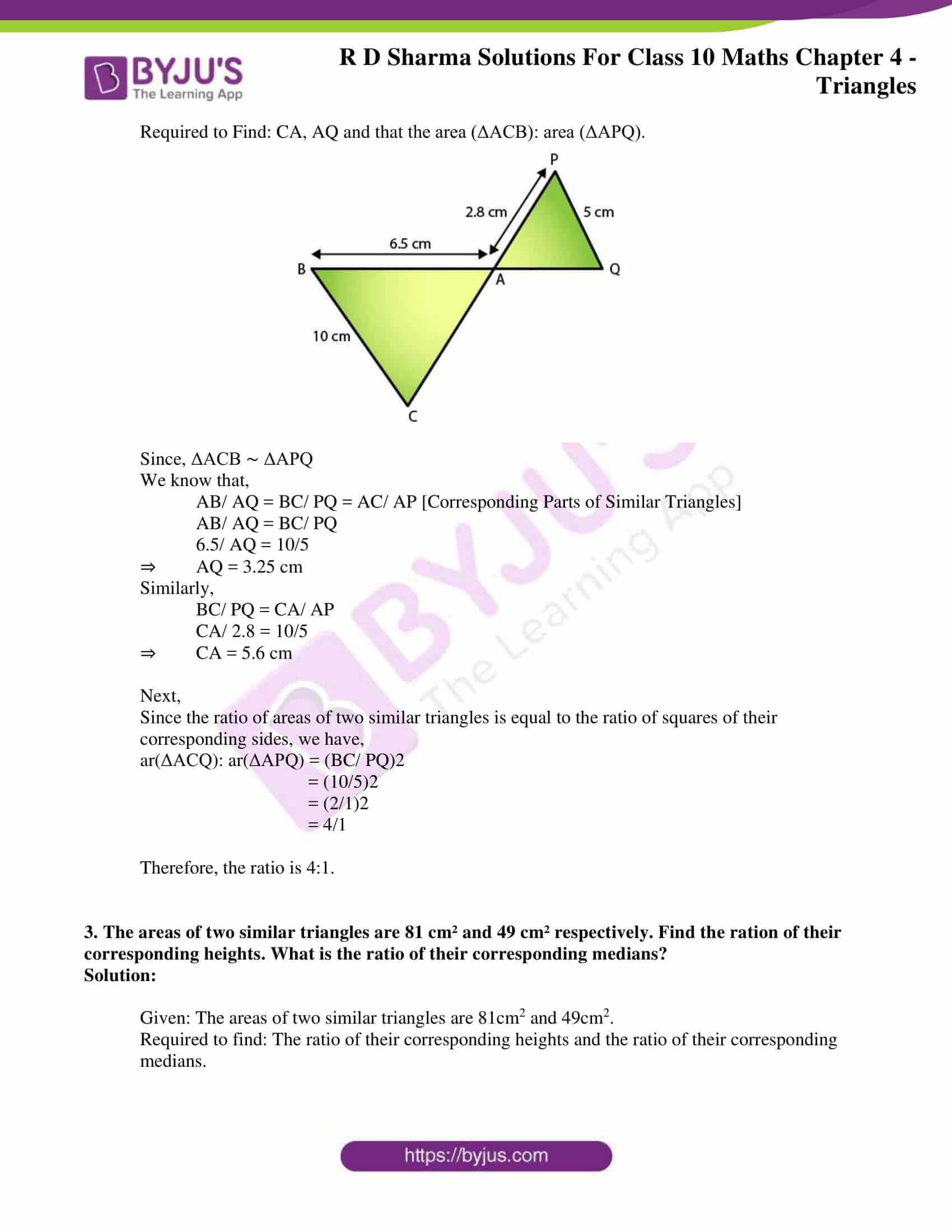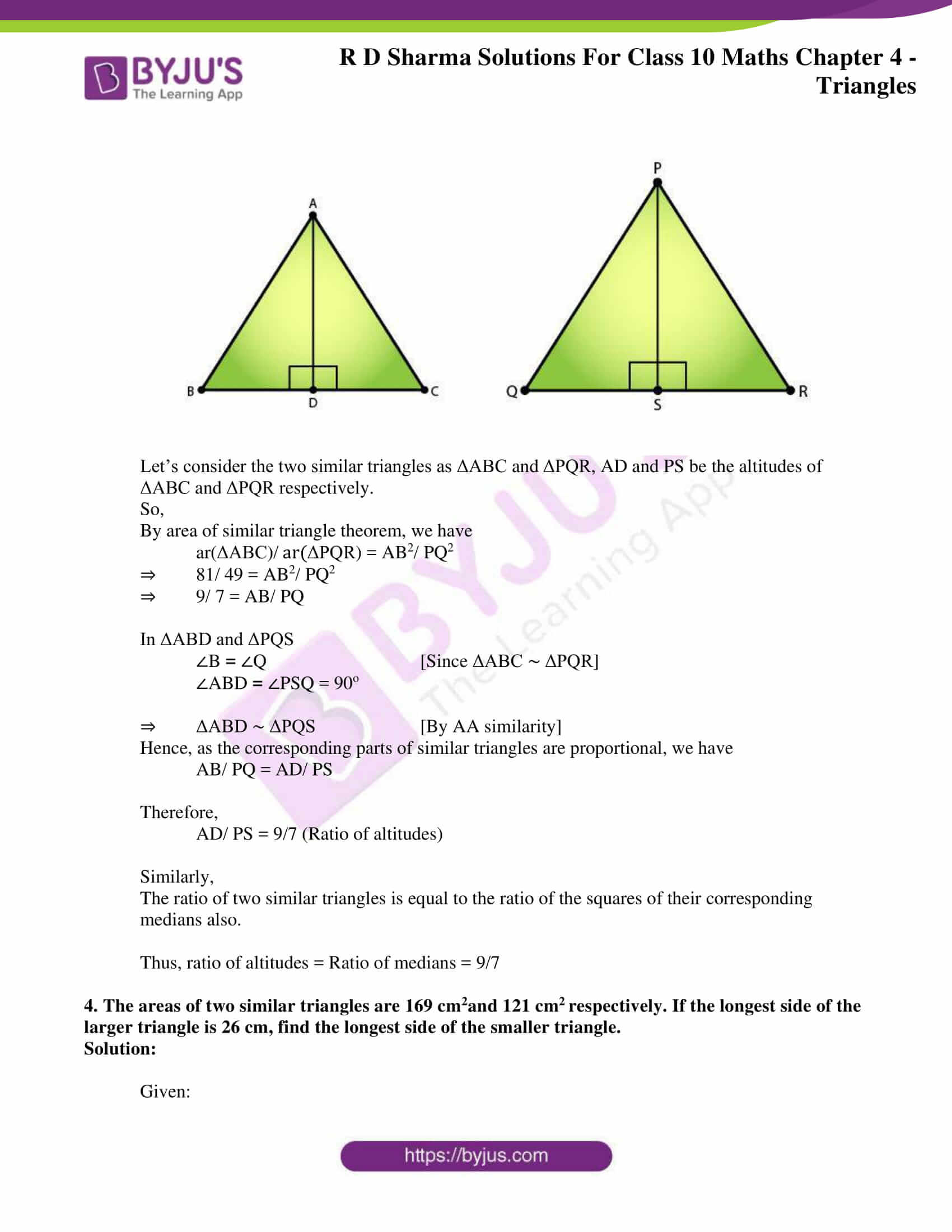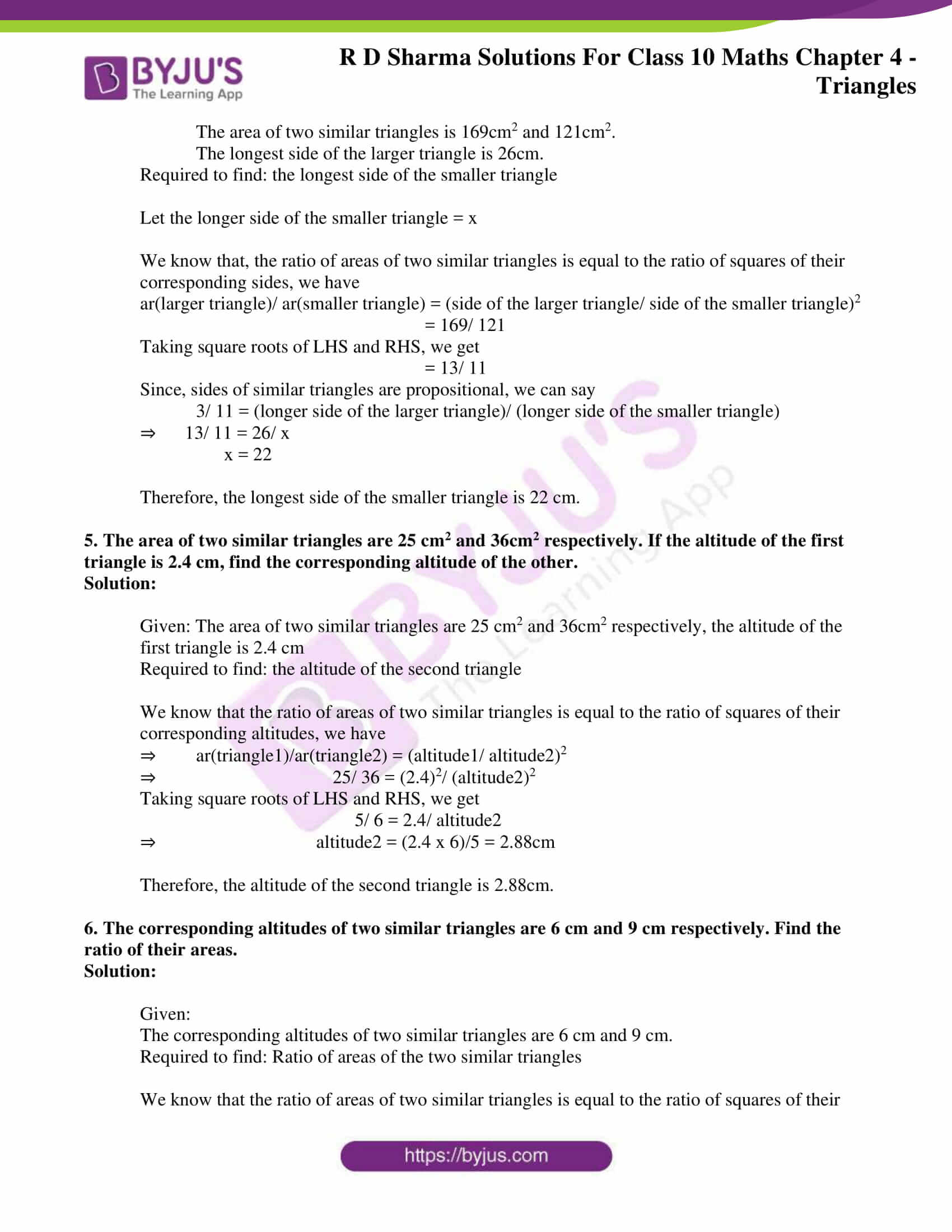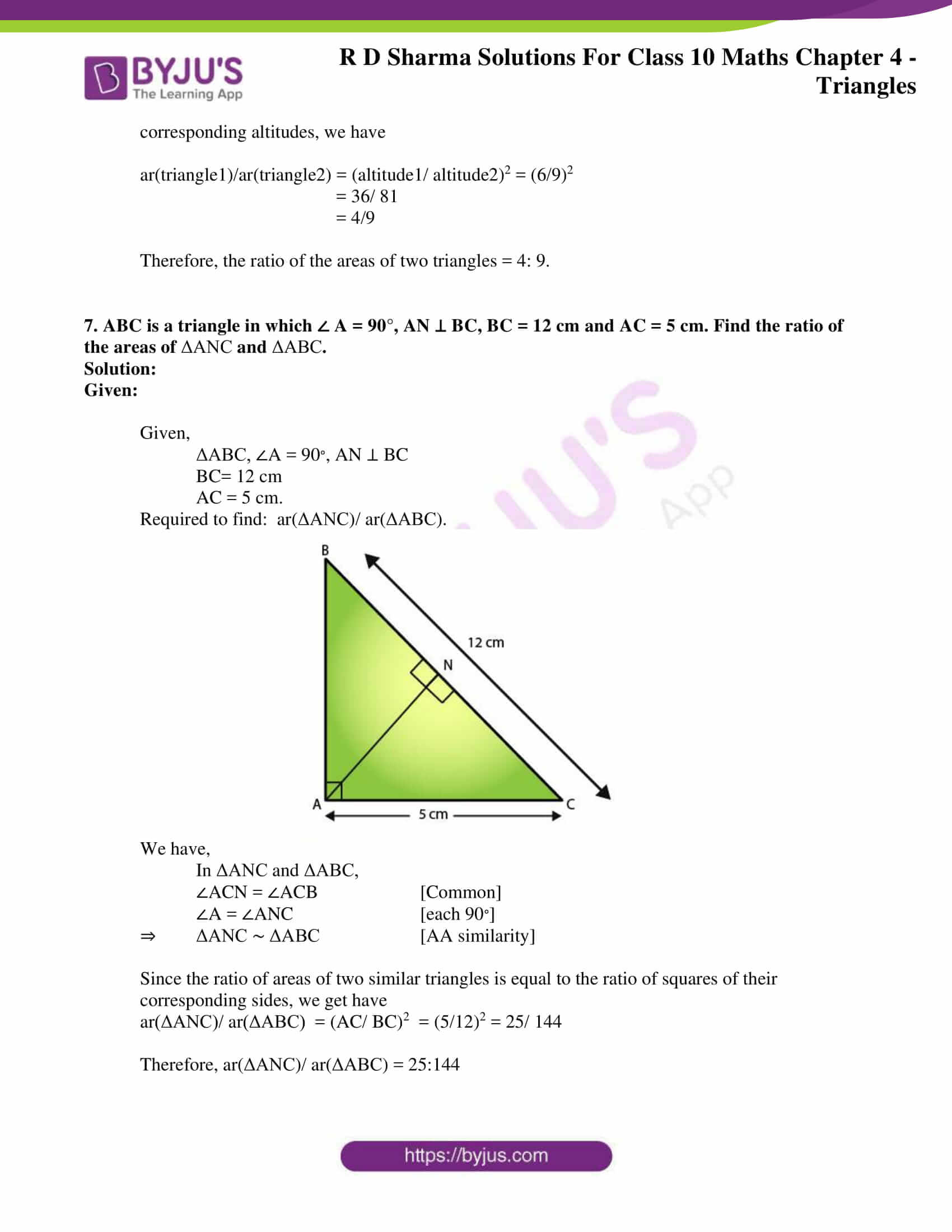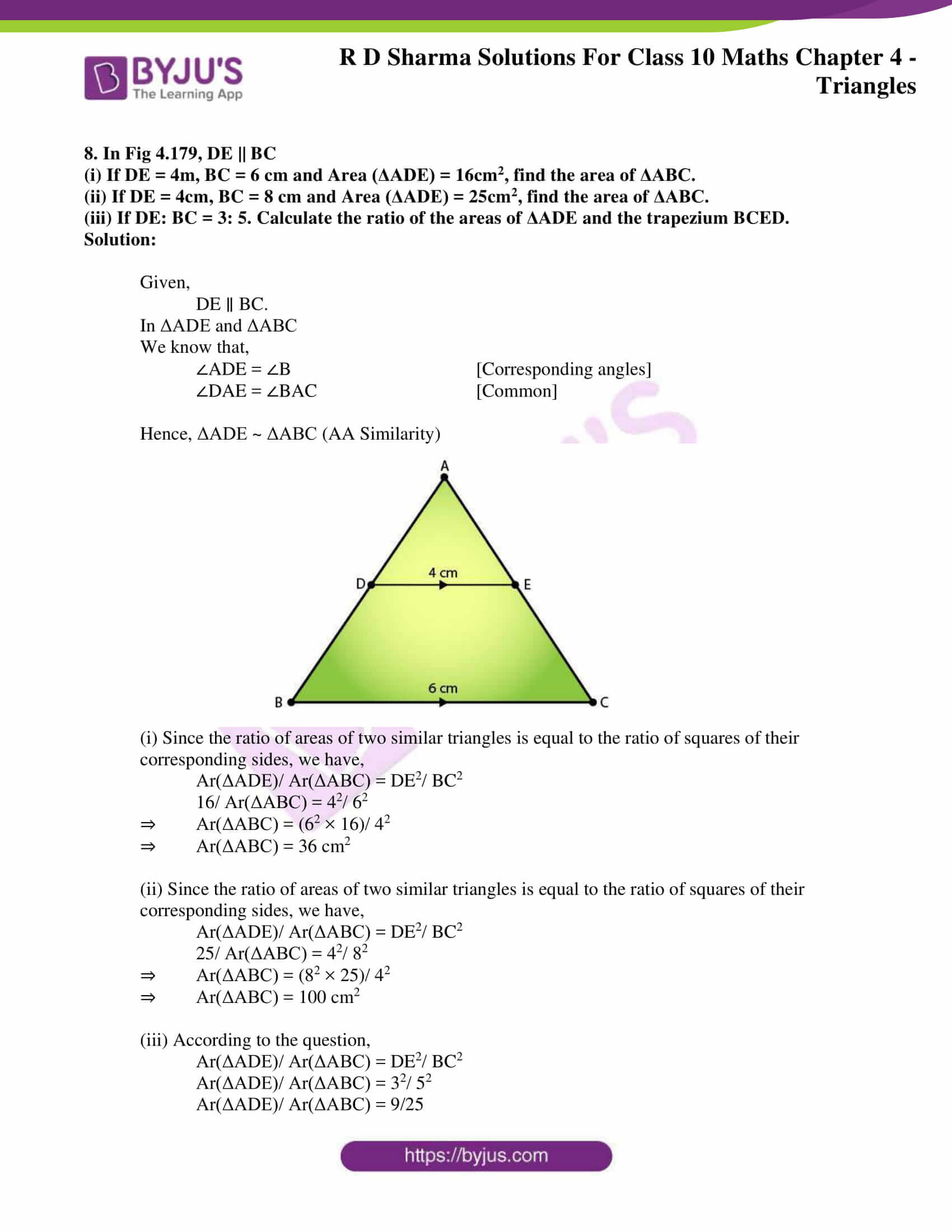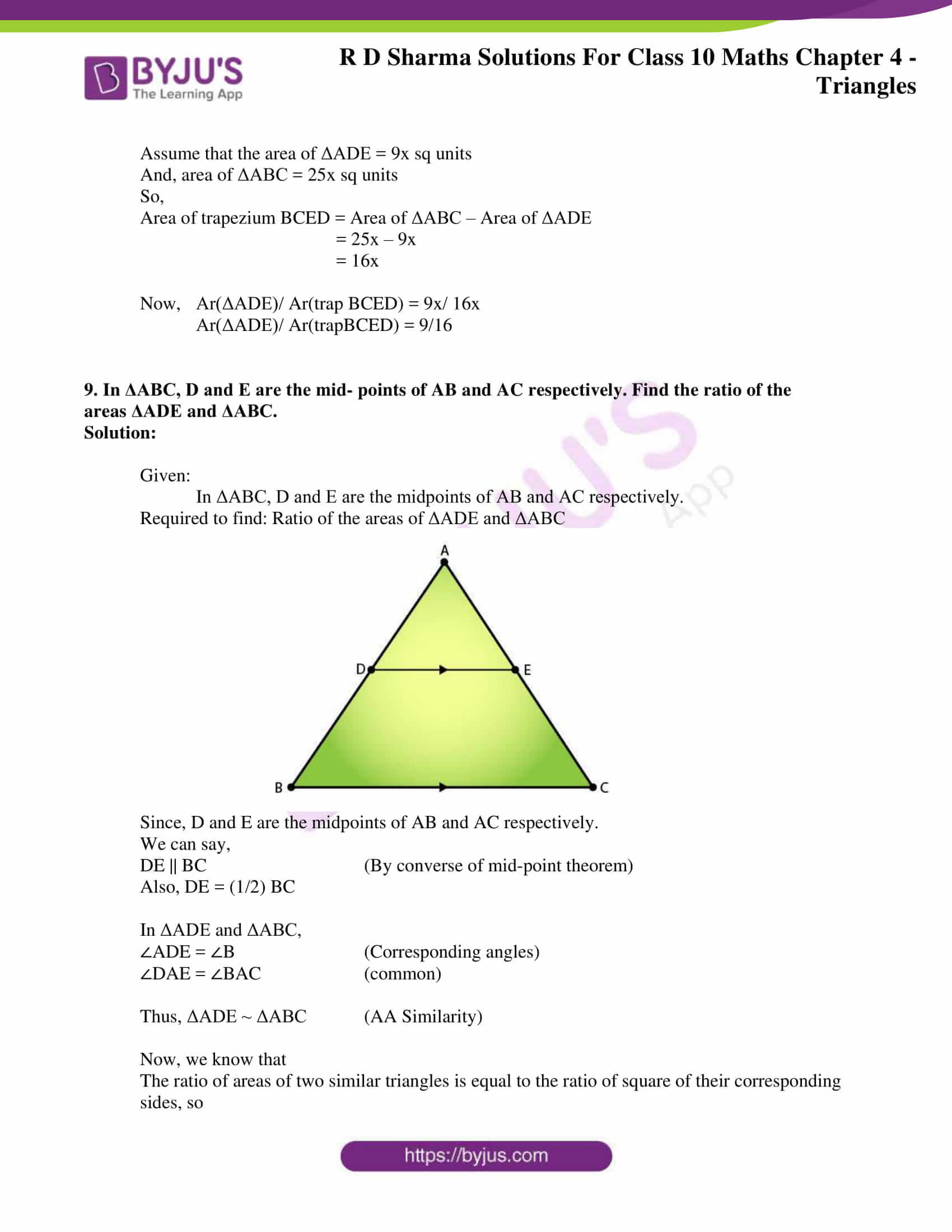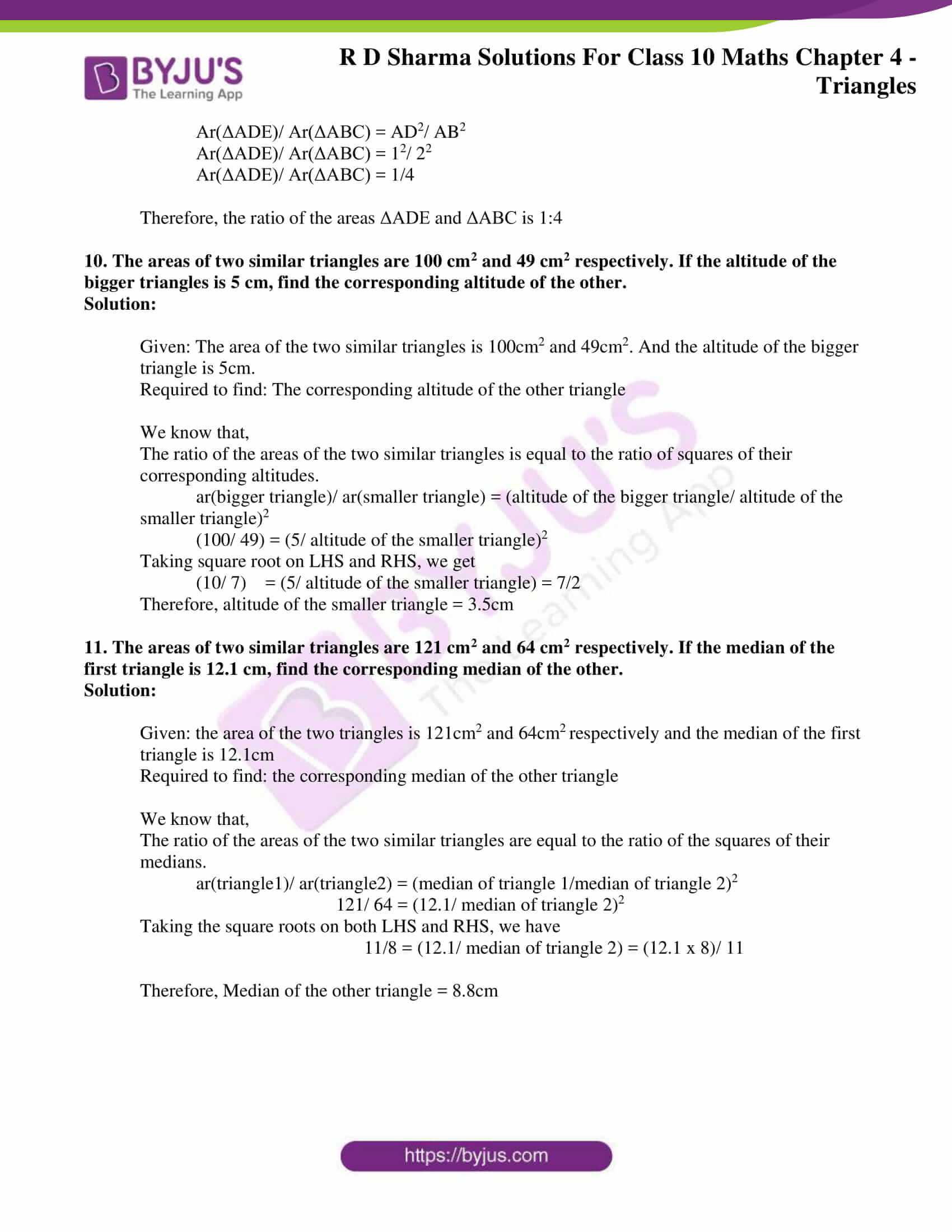### Access RD Sharma Solutions for Class 10 Maths Chapter 4 Triangles Exercise 4.6

1. Triangles ABC and DEF are similar.

(i) If area of (ΔABC) = 16 cm2, area (ΔDEF) = 25 cmand BC = 2.3 cm, find EF.

(ii) If area (ΔABC) = 9 cm2, area (ΔDEF) = 64 cmand DE = 5.1 cm, find AB.

(iii) If AC = 19 cm and DF = 8 cm, find the ratio of the area of two triangles.

(iv) If area of (ΔABC) = 36 cm2, area (ΔDEF) = 64 cmand DE = 6.2 cm, find AB.

(v) If AB = 1.2 cm and DE = 1.4 cm, find the ratio of the area of two triangles.

Solutions:

As we know that, the ratio of areas of two similar triangles is equal to the ratio of squares of their corresponding sides, we get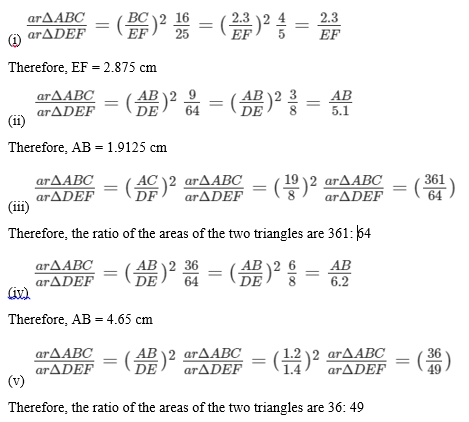2. In the fig 4.178, ΔACB ∼ ΔAPQ. If BC = 10 cm, PQ = 5 cm, BA = 6.5 cm, AP = 2.8 cm, find CA and AQ. Also, find the area (ΔACB): area (ΔAPQ).

Solution:

Given:

ΔACB is similar to ΔAPQ

BC = 10 cm

PQ = 5 cm

BA = 6.5 cm

AP = 2.8 cm

Required to Find: CA, AQ and that the area (ΔACB): area (ΔAPQ).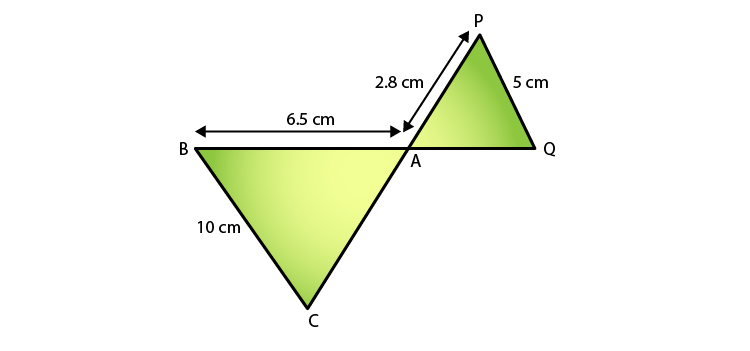Since, ΔACB ∼ ΔAPQ

We know that,

AB/ AQ = BC/ PQ = AC/ AP [Corresponding Parts of Similar Triangles]

AB/ AQ = BC/ PQ

6.5/ AQ = 10/5

⇒ AQ = 3.25 cm

Similarly,

BC/ PQ = CA/ AP

CA/ 2.8 = 10/5

⇒ CA = 5.6 cm

Next,

Since the ratio of areas of two similar triangles is equal to the ratio of squares of their corresponding sides, we have,

ar(ΔACQ): ar(ΔAPQ) = (BC/ PQ)2

= (10/5)2

= (2/1)2

= 4/1

Therefore, the ratio is 4:1.

3. The areas of two similar triangles are 81 cm² and 49 cm² respectively. Find the ration of their corresponding heights. What is the ratio of their corresponding medians?

Solution:

Given: The areas of two similar triangles are 81cm2 and 49cm2.

Required to find: The ratio of their corresponding heights and the ratio of their corresponding medians.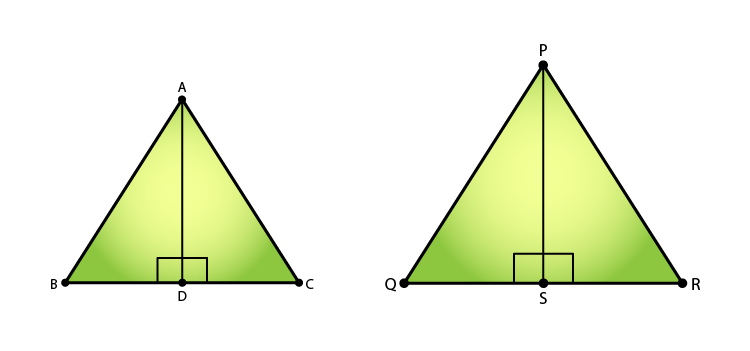Let’s consider the two similar triangles as ΔABC and ΔPQR, AD and PS be the altitudes of ΔABC and ΔPQR respectively.

So,

By area of similar triangle theorem, we have

ar(ΔABC)/ ar(ΔPQR) = AB2/ PQ2

⇒ 81/ 49 = AB2/ PQ2

⇒ 9/ 7 = AB/ PQ

In ΔABD and ΔPQS

∠B = ∠Q [Since ΔABC ∼ ΔPQR]

∠ABD = ∠PSQ = 90o

⇒ ΔABD ∼ ΔPQS [By AA similarity]

Hence, as the corresponding parts of similar triangles are proportional, we have

Therefore,

AD/ PS = 9/7 (Ratio of altitudes)

Similarly,

The ratio of two similar triangles is equal to the ratio of the squares of their corresponding medians also.

Thus, ratio of altitudes = Ratio of medians = 9/7

4. The areas of two similar triangles are 169 cm2and 121 cm2 respectively. If the longest side of the larger triangle is 26 cm, find the longest side of the smaller triangle.

Solution:

Given:

The area of two similar triangles is 169cm2 and 121cm2.

The longest side of the larger triangle is 26cm.

Required to find: the longest side of the smaller triangle

Let the longer side of the smaller triangle = x

We know that, the ratio of areas of two similar triangles is equal to the ratio of squares of their corresponding sides, we have

ar(larger triangle)/ ar(smaller triangle) = (side of the larger triangle/ side of the smaller triangle)2

= 169/ 121

Taking square roots of LHS and RHS, we get

= 13/ 11

Since, sides of similar triangles are propositional, we can say

3/ 11 = (longer side of the larger triangle)/ (longer side of the smaller triangle)

⇒ 13/ 11 = 26/ x

x = 22

Therefore, the longest side of the smaller triangle is 22 cm.

5. The area of two similar triangles are 25 cm2 and 36cm2 respectively. If the altitude of the first triangle is 2.4 cm, find the corresponding altitude of the other.

Solution:

Given: The area of two similar triangles are 25 cm2 and 36cm2 respectively, the altitude of the first triangle is 2.4 cm

Required to find: the altitude of the second triangle

We know that the ratio of areas of two similar triangles is equal to the ratio of squares of their corresponding altitudes, we have

⇒ ar(triangle1)/ar(triangle2) = (altitude1/ altitude2)2

⇒ 25/ 36 = (2.4)2/ (altitude2)2

Taking square roots of LHS and RHS, we get

5/ 6 = 2.4/ altitude2

⇒ altitude2 = (2.4 x 6)/5 = 2.88cm

Therefore, the altitude of the second triangle is 2.88cm.

6. The corresponding altitudes of two similar triangles are 6 cm and 9 cm respectively. Find the ratio of their areas.

Solution:

Given:

The corresponding altitudes of two similar triangles are 6 cm and 9 cm.

Required to find: Ratio of areas of the two similar triangles

We know that the ratio of areas of two similar triangles is equal to the ratio of squares of their corresponding altitudes, we have

ar(triangle1)/ar(triangle2) = (altitude1/ altitude2)2 = (6/9)2

= 36/ 81

= 4/9

Therefore, the ratio of the areas of two triangles = 4: 9.

7. ABC is a triangle in which ∠ A = 90°, AN ⊥ BC, BC = 12 cm and AC = 5 cm. Find the ratio of the areas of ΔANC and ΔABC.

Solution:

Given:

Given,

ΔABC, ∠A = 90, AN ⊥ BC

BC= 12 cm

AC = 5 cm.

Required to find: ar(ΔANC)/ ar(ΔABC).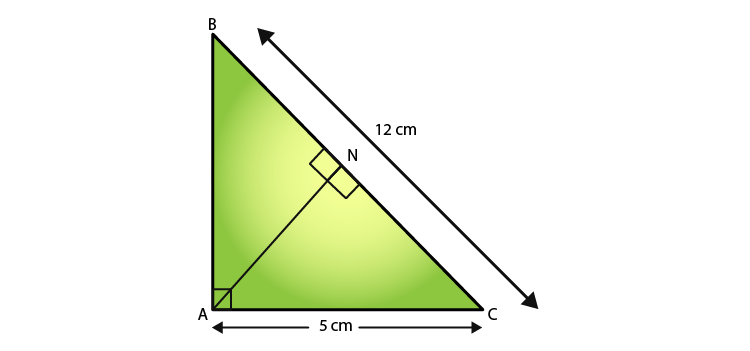We have,

In ΔANC and ΔABC,

∠ACN = ∠ACB  [Common]

∠A = ∠ANC  [each 90]

⇒ ΔANC ∼ ΔABC  [AA similarity]

Since the ratio of areas of two similar triangles is equal to the ratio of squares of their corresponding sides, we get have

ar(ΔANC)/ ar(ΔABC) = (AC/ BC)2 = (5/12)2 = 25/ 144

Therefore, ar(ΔANC)/ ar(ΔABC) = 25:144

8. In Fig 4.179, DE || BC

(i) If DE = 4m, BC = 6 cm and Area (ΔADE) = 16cm2, find the area of ΔABC.

(ii) If DE = 4cm, BC = 8 cm and Area (ΔADE) = 25cm2, find the area of ΔABC.

(iii) If DE: BC = 3: 5. Calculate the ratio of the areas of ΔADE and the trapezium BCED.

Solution:

Given,

DE ∥ BC.

We know that,

∠DAE = ∠BAC  [Common]

Hence, ΔADE ~ ΔABC (AA Similarity)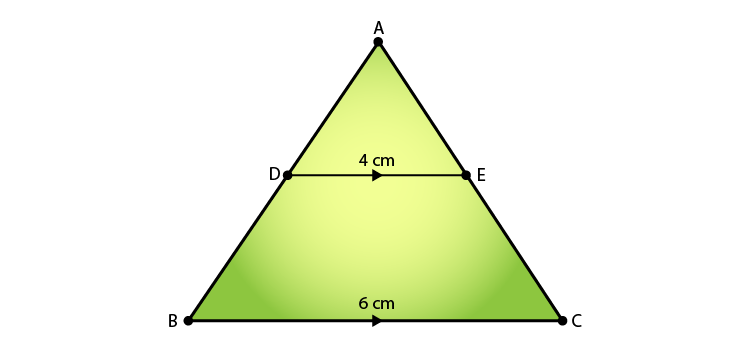(i) Since the ratio of areas of two similar triangles is equal to the ratio of squares of their corresponding sides, we have,

16/ Ar(ΔABC) = 42/ 62

⇒ Ar(ΔABC) = (62 × 16)/ 42

⇒ Ar(ΔABC) = 36 cm2

(ii) Since the ratio of areas of two similar triangles is equal to the ratio of squares of their corresponding sides, we have,

25/ Ar(ΔABC) = 42/ 82

⇒ Ar(ΔABC) = (82 × 25)/ 42

⇒ Ar(ΔABC) = 100 cm2

(iii) According to the question,

Assume that the area of ΔADE = 9x sq units

And, area of ΔABC = 25x sq units

So,

Area of trapezium BCED = Area of ΔABC – Area of ΔADE

= 25x – 9x

= 16x

Now,  Ar(ΔADE)/ Ar(trap BCED) = 9x/ 16x

9. In ΔABC, D and E are the mid- points of AB and AC respectively. Find the ratio of the areas ΔADE and ΔABC.

Solution:

Given:

In ΔABC, D and E are the midpoints of AB and AC respectively.

Required to find: Ratio of the areas of ΔADE and ΔABC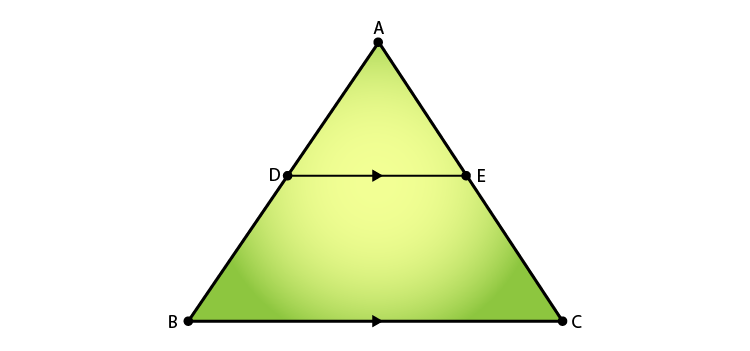Since, D and E are the midpoints of AB and AC respectively.

We can say,

DE || BC (By converse of mid-point theorem)

Also, DE = (1/2) BC

∠DAE = ∠BAC  (common)

Thus, ΔADE ~ ΔABC  (AA Similarity)

Now, we know that

The ratio of areas of two similar triangles is equal to the ratio of square of their corresponding sides, so

Therefore, the ratio of the areas ΔADE and ΔABC is 1:4

10. The areas of two similar triangles are 100 cm2 and 49 cm2 respectively. If the altitude of the bigger triangles is 5 cm, find the corresponding altitude of the other.

Solution:

Given: The area of the two similar triangles is 100cm2 and 49cm2. And the altitude of the bigger triangle is 5cm.

Required to find: The corresponding altitude of the other triangle

We know that,

The ratio of the areas of the two similar triangles is equal to the ratio of squares of their corresponding altitudes.

ar(bigger triangle)/ ar(smaller triangle) = (altitude of the bigger triangle/ altitude of the smaller triangle)2

(100/ 49) = (5/ altitude of the smaller triangle)2

Taking square root on LHS and RHS, we get

(10/ 7) = (5/ altitude of the smaller triangle) = 7/2

Therefore, altitude of the smaller triangle = 3.5cm

11. The areas of two similar triangles are 121 cm2 and 64 cm2 respectively. If the median of the first triangle is 12.1 cm, find the corresponding median of the other.

Solution:

Given: the area of the two triangles is 121cm2 and 64cm2 respectively and the median of the first triangle is 12.1cm

Required to find: the corresponding median of the other triangle

We know that,

The ratio of the areas of the two similar triangles are equal to the ratio of the squares of their medians.

ar(triangle1)/ ar(triangle2) = (median of triangle 1/median of triangle 2)2

121/ 64 = (12.1/ median of triangle 2)2

Taking the square roots on both LHS and RHS, we have

11/8 = (12.1/ median of triangle 2) = (12.1 x 8)/ 11

Therefore, Median of the other triangle = 8.8cm